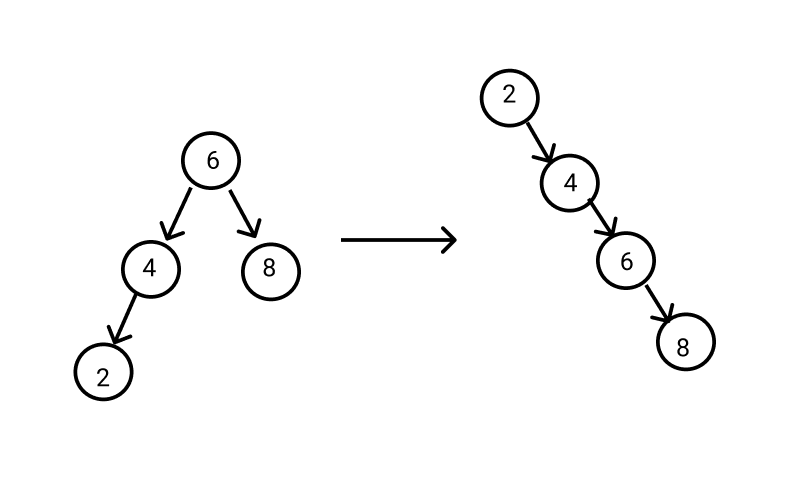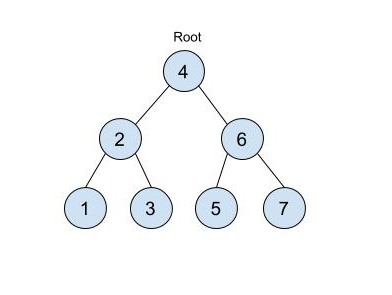# Increasing Order Search Tree

Posted: 10 Mar, 2021
Difficulty: Easy

## PROBLEM STATEMENT

#### Ninja has been given a binary search tree. Ninja has to rearrange the tree such that:

``````1. The leftmost node of the binary search tree becomes the root of the tree.
2. Every node has no left child.
3. Every node has only one right child.
``````
##### For example:#### As Ninja is busy, he asks you for help. Can you help Ninja to rearrange the binary search tree?

##### Input Format:
``````The first line of input contains an integer 'T' representing the number of test cases. Then the test cases follow.

The next line of each test case contains elements in the level order form. The line consists of values of nodes separated by a single space. In case a node is null, we take -1 on its place.

For example, the input for the tree depicted in the below image would be :
```````````` 4
2 6
1 3 5 7
-1 -1 -1 -1 -1 -1
``````
##### Explanation :
``````Level 1 :
The root node of the tree is 4

Level 2 :
Left child of 4 = 2
Right child of 4 = 6

Level 3 :
Left child of 2 = 1
Right child of 2 = 3
Left child of 6 = 5
Right child of 6 = 7

Level 4 :
Left child of 1 = null (-1)
Right child of 1 = null (-1)
Left child of 3 = null (-1)
Right child of 3 = null (-1)
Left child of 5 = null (-1)
Right child of 5 = null (-1)
Left child of 7 = null (-1)
Right child of 7 = null (-1)

The first not-null node(of the previous level) is treated as the parent of the first two nodes of the current level. The second not-null node (of the previous level) is treated as the parent node for the next two nodes of the current level and so on.
The input ends when all nodes at the last level are null(-1).
``````
##### Note :
``````The above format was just to provide clarity on how the input is formed for a given tree.

The sequence will be put together in a single line separated by a single space. Hence, for the above-depicted tree, the input will be given as:

4 2 6 1 3 5 7 -1 -1 -1 -1 -1 -1
``````
##### Output Format :
``````For each test case, print the tree after the rearrangement in level order.

Print the output of each test case in a separate line.
``````
##### Note:
``````You do not need to print anything; it has already been taken care of. Just implement the given function.
``````
##### Constraints :
``````1 <= T <= 10
1 <= N <= 10000
-100000 <= data <= 100000

Where ‘T’ denotes the number of test cases, ‘N’ denotes the number of nodes in the binary tree and ‘data’ represents the value of each node of the binary search tree.

Time Limit: 1 second
``````Approach 1

We know the inorder traversal of the binary search tree is increasing in order. So first we apply the inorder traversal and store all the nodes in a list/vector ‘ALL_NODES’. After that, we iterate through the list/vector ‘ALL_NODES’  and add every node to the right of the tree, and then return the root.

Here is the algorithm:

1. We declare a list/vector ‘ALL_NODES’ in which we store all nodes of the given tree.
2. Apply inorder traversal on the tree and store all nodes into the ‘ALL_NODES’.
3. We declare a node ‘ANS_ROOT’ and ‘CURR_NODE’ which represent the root of the rearranged tree and the current node of the tree.
4. We run a loop for ‘i’ = ‘1’ to the length of ‘ALL_NODES’:
• Add a new node to the right of ‘CURR_NODE’ .
• Now ‘CURR_NODE’ is right of the ‘CURR_NODE’.
5. Return ‘ANS_ROOT’.